#### Learning Objectives

Upon completion of this exercise, you should be able to:

• Define trituration and geometric dilution.
• Demonstrate techniques of geometric dilution (e.g., preparation of powder triturations) and serial dilution (e.g., preparation of liquid aliquots).
• Describe the techniques and perform the calculations for obtaining a quantity of a substance less than the L.W.Q. for a solid dosage form (i.e. trituration method), and a liquid dosage form (i.e. liquid aliquot method).

#### Trituration Method

A trituration is a dilution of a potent drug powder with an inert diluent powder, usually lactose, in a definite proportion by weight. We can then remove a weighable portion (aliquot) of the mixture containing the desired quantity of substance to maintain an acceptable range of accuracy.

Using ratio and proportion, we can determine the weight of drug and lactose required to make the trituration as well as the weight of the aliquot to be used to fill the prescription.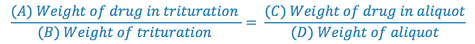Keep in mind that any substance that must be weighed on the balance drug (A), lactose (B-A), and aliquot (D) must be at least 120 mg.

Lactose is the most commonly used diluent in solid dosage form pharmaceuticals such as powders, tablets and capsules. Some of the reasons included its extremely low incidence of side effects, ready availability, and low cost.

Example: Make 10 capsules, each containing 0.6 mg Atropine Sulfate and each weighing a total of 200 mg.

### Make Trituration

1. Total weight atropine sulfate required (this is C in the proportion)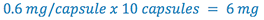2. Select some multiple of 6 mg that can be weighed with the desired degree of accuracy. (this is A in the proportion) (e.g. select multiple= 20)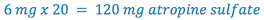3. Select the size of the aliquot portion to be taken to contain the 6 mg of drug. (this is D in the proportion)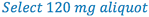4. Since we now have A, C, and D in our formula, we can solve for B (trituration weight).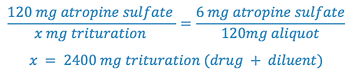[Note: this should be the multiple (20) x the weight of the aliquot (120 mg)]

5. Determine the weight of diluent (lactose) required to prepare the trituration by subtracting the weight of atropine sulfate from the trituration weight.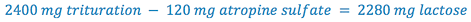6. Fill Rx:

Determine the weight of lactose which must be added to the aliquot portion of trituration to complete the prescription, so that each capsule weighs 200 mg.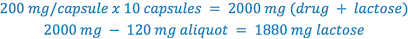After the necessary calculations are completed, the trituration is prepared using the following steps:

1. weigh a suitable amount of drug (at least 120 mg), and reduce it to a fine powder in a mortar (i.e., triturate it);
2. weigh a suitable amount of diluent;
3. mix the drug and diluent by geometric dilution and;
4. weigh an aliquot of the trituration containing the desired weight of drug.

View a video demonstration on geometric dilution

Hence the compounding procedure for this product is as follows:

• Weigh 120 mg atropine sulfate and triturate to a fine powder
• Weigh 2280 mg lactose
• Mix atropine sulfate and lactose by geometric dilution
• Remove 120 mg of the trituration (contains 6 mg atropine sulfate)
(Hint: save left over trituration for refills)
• Weigh 1880 mg lactose and mix with 120 mg aliquot by geometric dilution
• Prepare capsules weighing 200 mg each

#### Liquid Aliquot Method

This technique is similar to the trituration method but employs liquid rather than solid diluents. It is used only when the product to be dispensed is a liquid. A weighable quantity of drug is dissolved in a calculated volume of a suitable solvent, usually water or ethanol. Then, an aliquot of the solution containing the desired amount of drug is removed. Both the total volume of solution and the aliquot volume should be such that they are easily and accurately measured (preferably whole number multiples of 5 ml).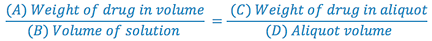e.g. Prepare 100 ml of a solution containing 0.2 mg/ml clonidine.

(Need 100 ml x 0.2 mg/ml = 20 mg clonidine)

1. Select a suitable solvent (based on drug solubility and stability, route of administration, and potential toxicity of the solvent).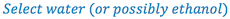2. Select the aliquot volume (D) in which the desired amount of drug(C) will be contained. This establishes the concentration of the solution to be prepared. Volume must be large enough that concentration does not exceed the solubility of the drug, but small enough that it does not exceed the total volume of the prescription.

(Hint: check Remington’s or Merck Index for solubility data, then pick a concentration that is less than this solubility)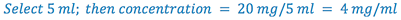3. Select weight of drug (120 mg) to prepare the solution. Whenever practical, use the least weighable quantity so as to minimize waste. (This is A in the proportion)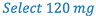4. Calculate the total volume (B) of solution to be prepared.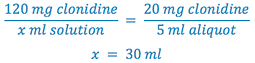5. Remove aliquot of solution and q.s. to total Rx volume with the appropriate vehicle.

Hence, we would:

• Weigh 120 mg clonidine
• Dissolve the clonidine in sufficient water to make 30 ml (Why not dissolve in 30 ml of ethanol?)
• Remove 5 ml aliquot
• q.s. with H2O to 100 ml to complete the prescription